## Wednesday, July 6, 2022

### Understanding the number of changes (of different types) in a stochastic character mapping analysis using phytools

Today, an R phylogenetics user asked the question:

“I am interested in determining how many times a trait was gained across a phylogeny. So I simulated the evolution of the trait (using simmap in phytools) and I can look at the mean number of gains across in the simulations, which in my case was ~20. However, I would also like to be able to point at the nodes where the trait was likely gained on my tree. For this, I simply rounded the posterior probability at each node to either 0 or 1. However, this only gives me 18 nodes where the trait was likely gained. I think I can see why there is a difference between those numbers; although there are 20 gains on average, since those gains aren't always going to occur on the same nodes, then there aren't going to be 20 nodes with a probability of gaining the trait >0.5. My question is (1) is my logic right as to why that discrepancy exists, and (2) if I want to say 'this trait likely evolved X number of times', which is the more correct way to get that number? Should I report the mean/median (maybe with confidence intervals), or does it make more sense to look at the posterior probability at each node and get a number that way?”

OK.

So in stochastic character mapping, what we're doing is sampling plausible character histories that are consistent with our tip data, under an evolutionary model.

In phytools the default way to do this is to estimate a value for the transition matrix, Q, using likelihood, and the sampling trait histories with stochastic simulations given this value of Q that are consistent with our observed data. [This isn't the only way to do this in phytools. We could have also using MCMC to first sample Q from its posterior probability distribution, and then used these values to generate our character histories.]

This results in a distribution of trait changes on the tree, rather than a specific number of changes, as we might obtain using a non-statistical method such as parsimony. For a given number of changes, these different histories can also imply different sets of changes (in either direction) on the phylogeny.

Here I'm going to use a real example to give a sense of what I mean. These data are from Benun Sutton & Wilson (2019), and I also use them in my upcoming book with Luke Harmon.

``````library(phytools)
bonyfish.tree
``````
``````##
## Phylogenetic tree with 90 tips and 89 internal nodes.
##
## Tip labels:
##   Xenomystus_nigri, Chirocentrus_dorab, Talismania_bifurcata, Alepocephalus_tenebrosus, Misgurnus_bipartitus, Opsariichthys_bidens, ...
##
## Rooted; includes branch lengths.
``````
``````bonyfish.data<-read.csv(file="http://www.phytools.org/Rbook/7/bonyfish.csv",
row.names=1,stringsAsFactors=TRUE)
``````
``````##                          spawning_mode paternal_care
## Xenomystus_nigri                  pair          male
## Chirocentrus_dorab               group          none
## Talismania_bifurcata             group          none
## Alepocephalus_tenebrosus         group          none
## Misgurnus_bipartitus              pair          none
## Opsariichthys_bidens              pair          none
``````
``````plotTree.datamatrix(bonyfish.tree,X=bonyfish.data,yexp=1.1,fsize=0.5)
``````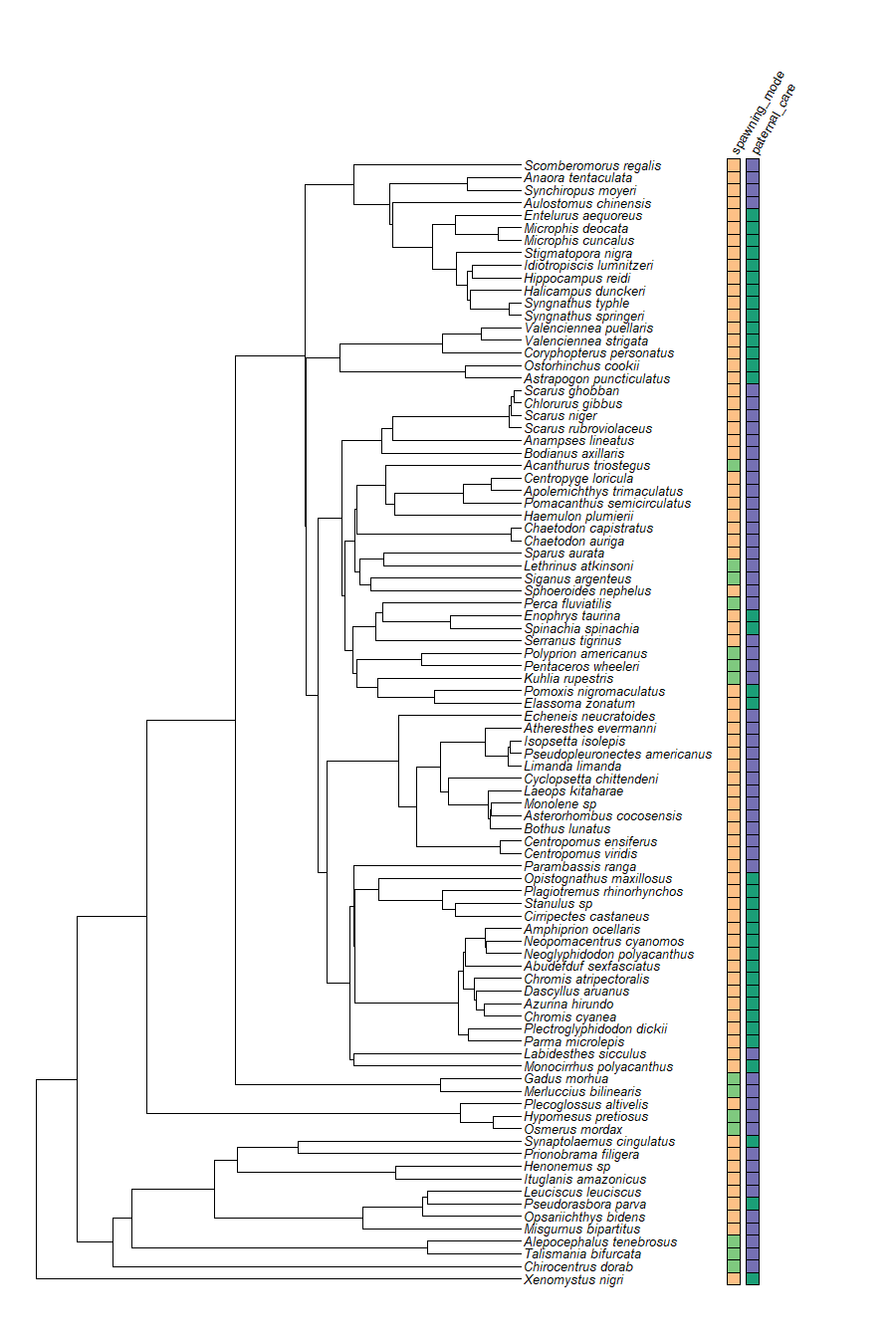We're going to analyze just one trait, spawning mode, so I'll pull that out to proceed.

``````spawning_mode<-setNames(bonyfish.data\$spawning_mode,
rownames(bonyfish.data))
``````

Now let's generate our stochastic character maps as follows.

``````smaps.spawning_mode<-make.simmap(bonyfish.tree,spawning_mode,
model="ARD",nsim=1000,pi="fitzjohn")
``````
``````## make.simmap is sampling character histories conditioned on
## the transition matrix
##
## Q =
##               group          pair
## group -0.0003478837  0.0003478837
## pair   0.0018750505 -0.0018750505
## (estimated using likelihood);
## and (mean) root node prior probabilities
## pi =
##       group        pair
## 0.002330267 0.997669733
``````
``````## Done.
``````
``````smaps.spawning_mode
``````
``````## 1000 phylogenetic trees with mapped discrete characters
``````

The first thing we can do is compute a posterior probability distribution on the number of changes of each type. This gives us a measure of both the expected number of changes, and the dispersion or variance of changes under our assumed model.

Doing this in phytools is easy. We just use the `density` method for our object class as follows.

``````dd<-density(smaps.spawning_mode)
dd
``````
``````##
## Distribution of changes from stochastic mapping:
##  group->pair     pair->group
##  Min.   :0   Min.   :9
##  Median :0   Median :10
##  Mean   :0.28    Mean   :10.3
##  Max.   :4   Max.   :12
##
## 95% HPD interval(group->pair): [0, 1]
## 95% HPD interval(pair->group): [10, 11]
``````
``````plot(dd,colors=c("black","orange"),alpha=0.5)
``````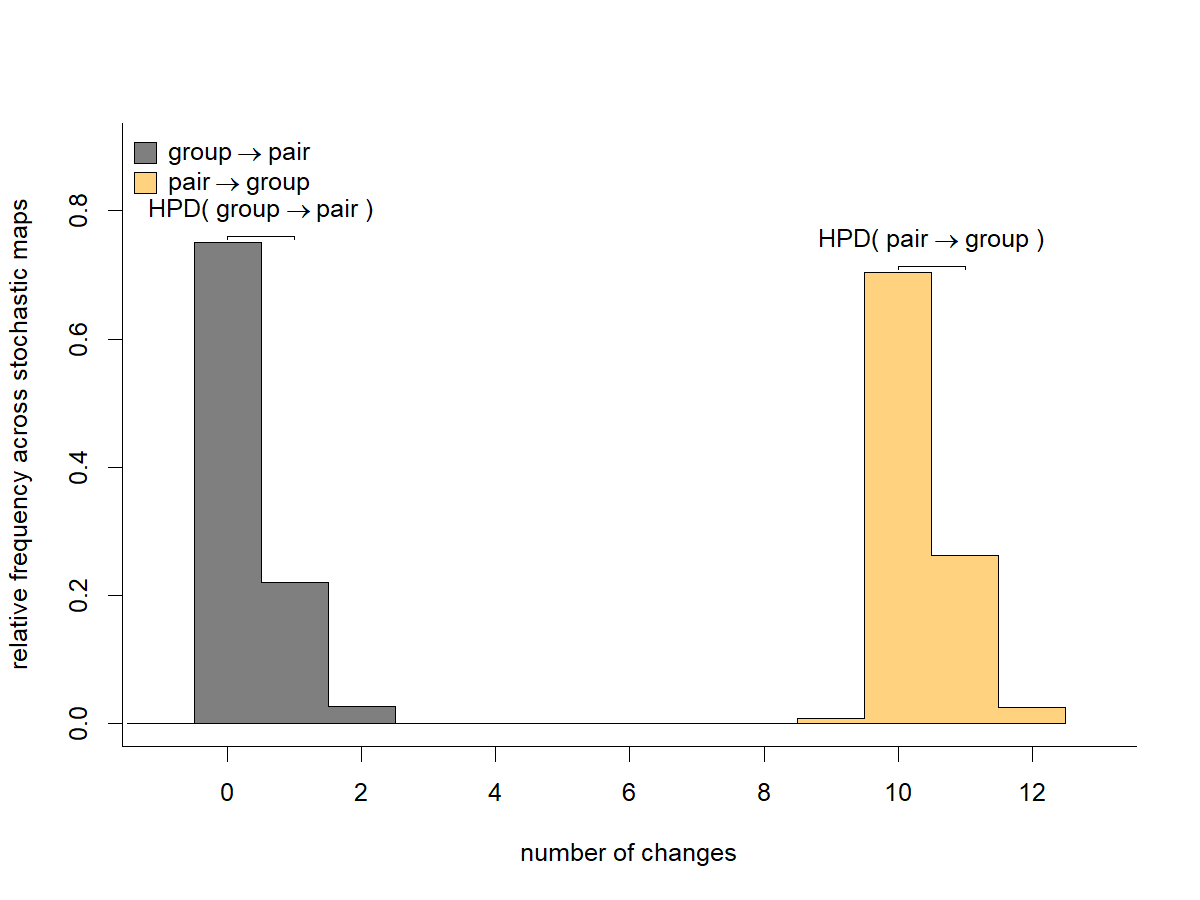This shows us that most of our stochastic character map histories show no, or very few, character changes from group to pair spawning, but between 9 and 12 changes from pair to group spawning.

To get the number of changes on each & every one of our 1,000 stochastic mapped trees, I'm going to run our `summary` method again, but this time separately on each `"simmap"` object in my posterior sample, instead of on the `"multiSimmap"` object as a whole.

``````ss<-lapply(smaps.spawning_mode,summary)
``````
``````## []
## 1 tree with a mapped discrete character with states:
##  group, pair
##
## tree has 10 changes between states
##
## changes are of the following types:
##       group pair
## group     0    0
## pair     10    0
##
## mean total time spent in each state is:
##            group         pair    total
## raw  679.2357201 5681.0307286 6360.266
## prop   0.1067936    0.8932064    1.000
##
##
## []
## 1 tree with a mapped discrete character with states:
##  group, pair
##
## tree has 10 changes between states
##
## changes are of the following types:
##       group pair
## group     0    0
## pair     10    0
##
## mean total time spent in each state is:
##            group         pair    total
## raw  818.6079802 5541.6584685 6360.266
## prop   0.1287066    0.8712934    1.000
``````

In this case, I see that the first two of my stochastic maps both have 10 changes, and that all of these changes are from pair to group spawning.

It's easy enough to get this tally for all 1,000 stochastically mapped trees.

``````n.changes<-t(sapply(ss,function(x) c(x\$Tr[2,1],x\$Tr[1,2])))
dimnames(n.changes)<-list(1:length(smaps.spawning_mode),
c("pair->group","group->pair"))
``````
``````##    pair->group group->pair
## 1           10           0
## 2           10           0
## 3           10           0
## 4           10           0
## 5           10           0
## 6           10           0
## 7           10           0
## 8           10           0
## 9           10           0
## 10          10           0
## 11          11           1
## 12          11           1
## 13          10           0
## 14          10           0
## 15          11           2
## 16          10           0
## 17          10           0
## 18          10           0
## 19          10           0
## 20          10           0
## 21          10           0
## 22          10           0
## 23          11           0
## 24          10           1
## 25          10           1
## 26          12           0
## 27          10           0
## 28          10           0
## 29          10           0
## 30          10           0
``````

Just inspecting the first 30 trees, we see that the most common history involves 10 changes from pair to group spawning, and zero changes in the reverse direction; however, our stochastic histories can sometimes involve as many as 12 changes, as well as a small number of changes in the opposite direction.

Let's graph some examples of these.

First, a stochastic character history with 10 “forward” (pair to group) changes and zero changes in the opposite direction.

``````## set colors
cols<-setNames(c("black","orange"),c("pair","group"))
## set map
i<-7
plot(smaps.spawning_mode[[i]],cols,ftype="i",fsize=0.6,
outline=TRUE,lwd=3,ylim=c(-5,Ntip(bonyfish.tree)))
markChanges(smaps.spawning_mode[[i]],
setNames(c("black","black"),names(cols)),cex=0.5,lwd=5)
markChanges(smaps.spawning_mode[[i]],cols,cex=0.5,lwd=3)
text(paste("Number of changes:",sum(n.changes[i,])),
x=mean(par()\$usr[1:2]),
y=-4,cex=1.5)
``````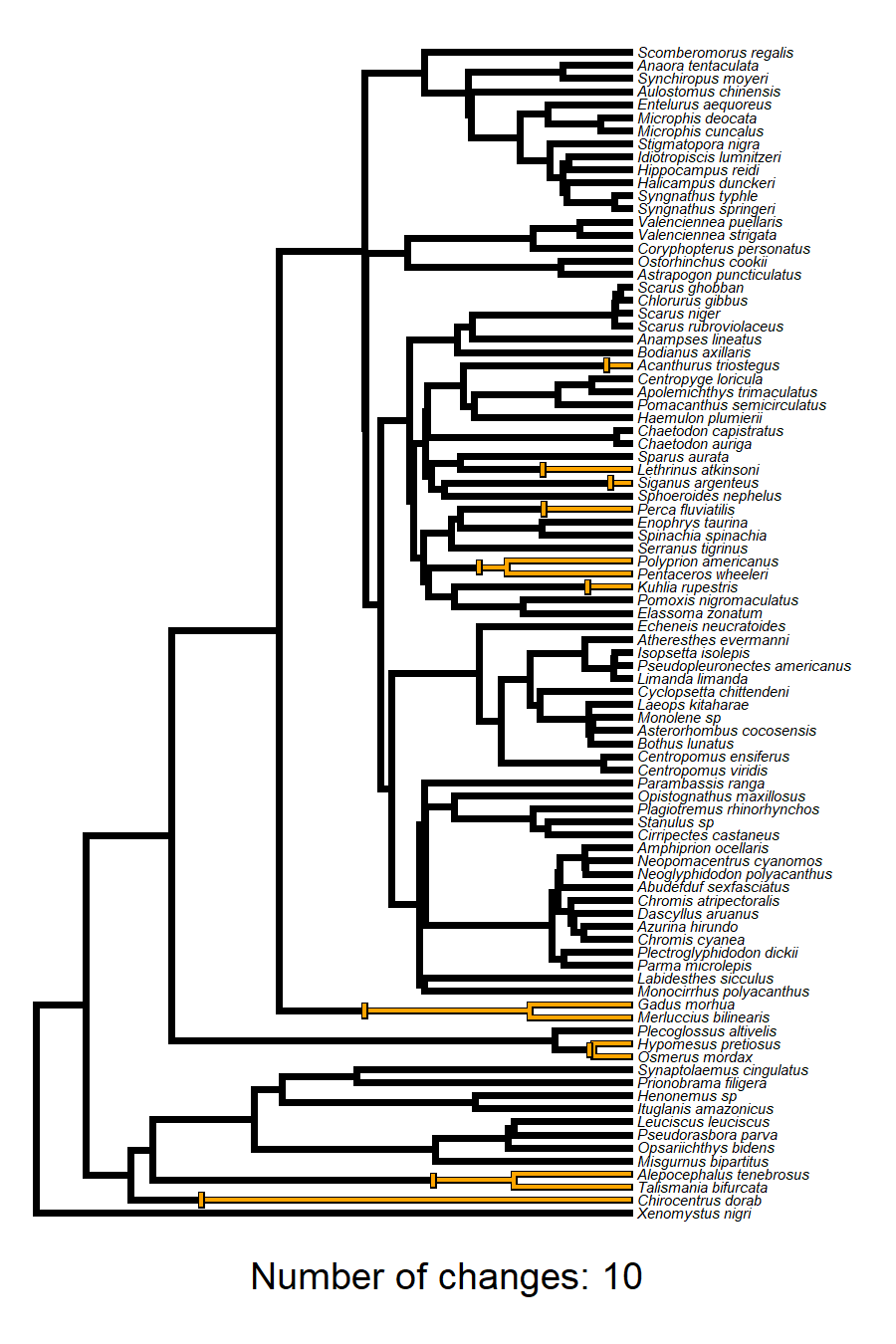Now, an example with 11 forward changes, and 2 reversals.

``````i<-749
plot(smaps.spawning_mode[[i]],cols,ftype="i",fsize=0.6,
outline=TRUE,lwd=3,ylim=c(-5,Ntip(bonyfish.tree)))
markChanges(smaps.spawning_mode[[i]],
setNames(c("black","black"),names(cols)),cex=0.5,lwd=5)
markChanges(smaps.spawning_mode[[i]],cols,cex=0.5,lwd=3)
text(paste("Number of changes:",sum(n.changes[i,])),
x=mean(par()\$usr[1:2]),
y=-4,cex=1.5)
``````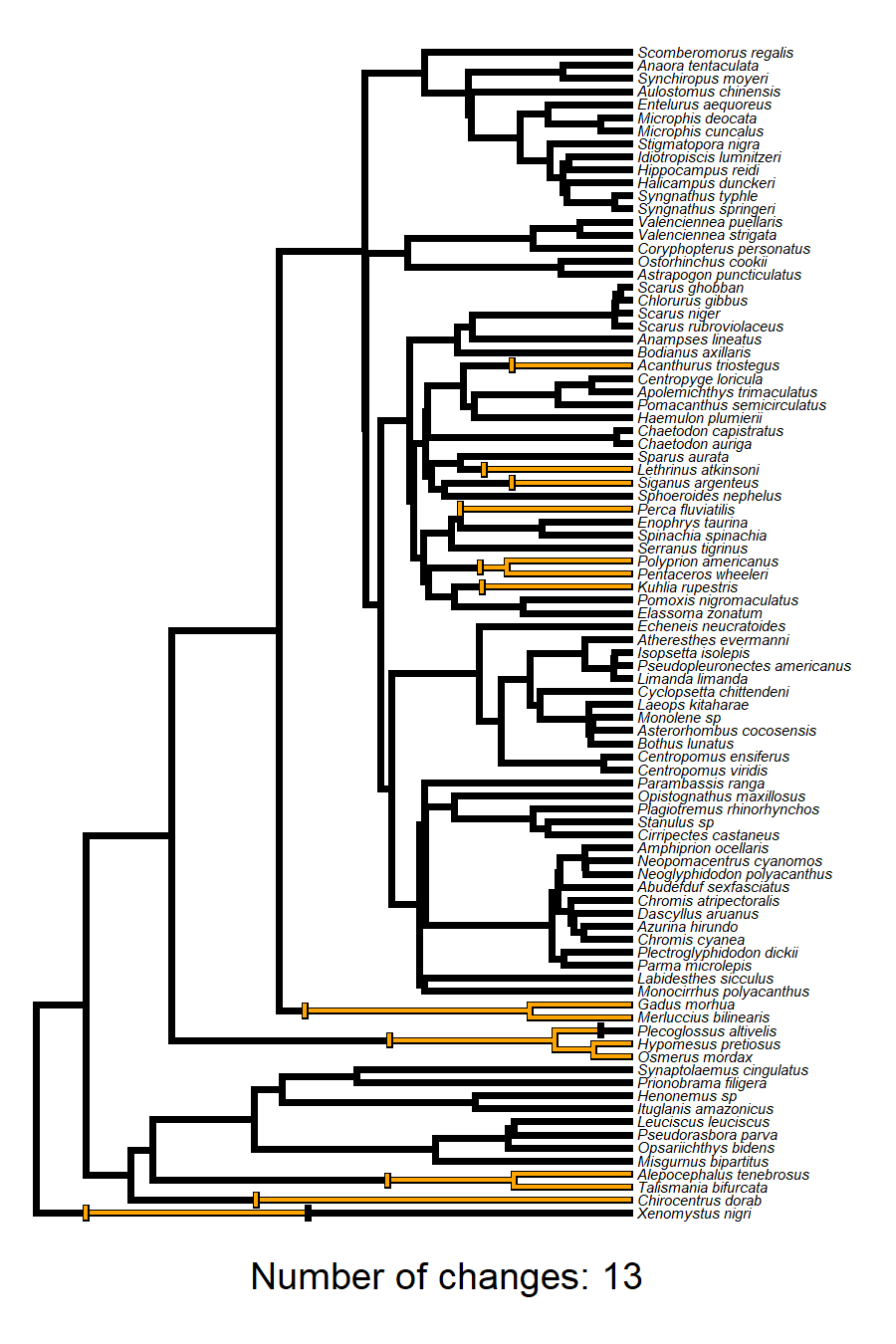Lastly, a rare example with only 9 forward changes (and one reversal).

``````i<-637
plot(smaps.spawning_mode[[i]],cols,ftype="i",fsize=0.6,
outline=TRUE,lwd=3,ylim=c(-5,Ntip(bonyfish.tree)))
markChanges(smaps.spawning_mode[[i]],
setNames(c("black","black"),names(cols)),cex=0.5,lwd=5)
markChanges(smaps.spawning_mode[[i]],cols,cex=0.5,lwd=3)
text(paste("Number of changes:",sum(n.changes[i,])),
x=mean(par()\$usr[1:2]),
y=-4,cex=1.5)
``````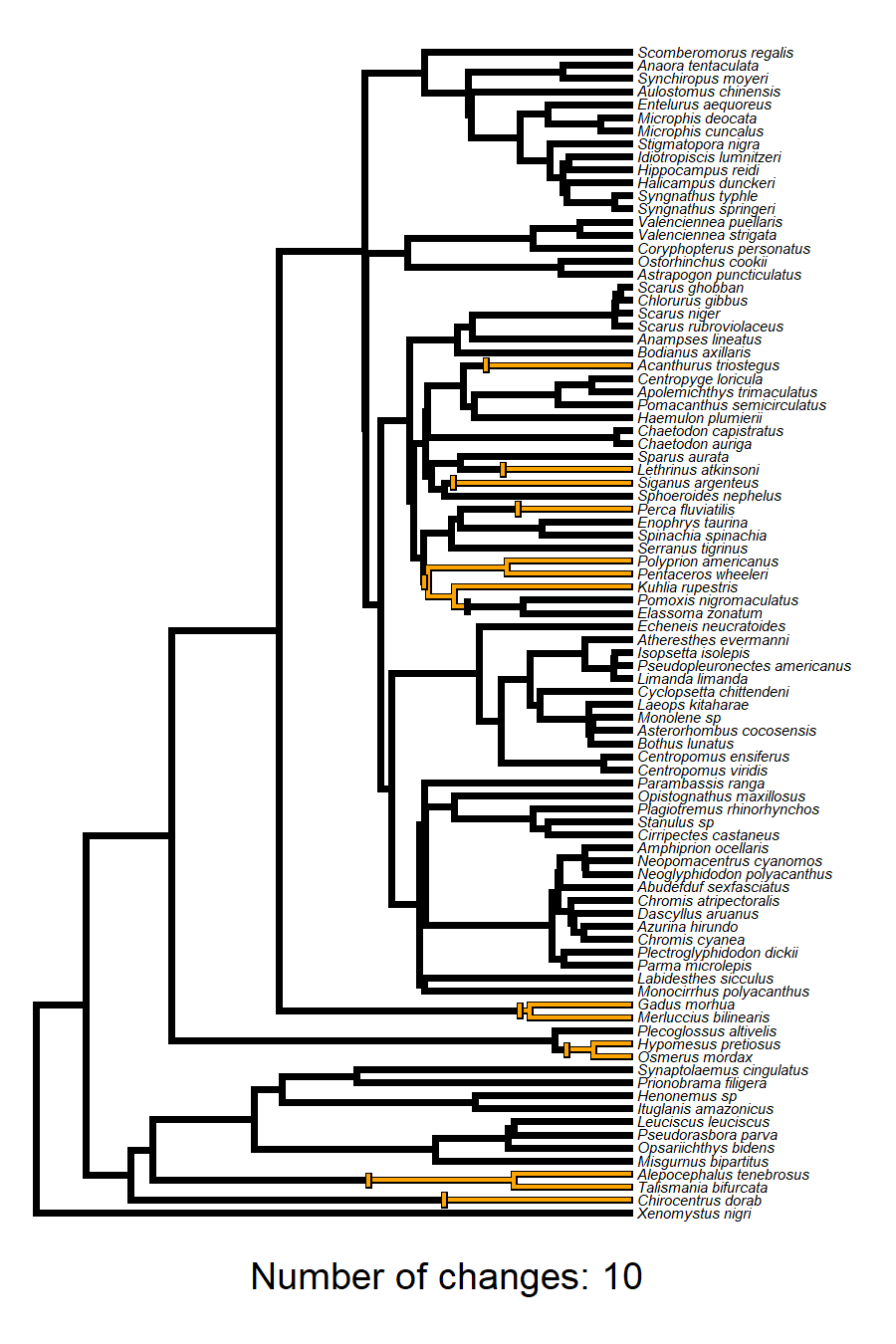All of these character histories are consistent with our tip data and have been sampled in proportion to their probability under a model of character evolution that we specified.

One interesting, but totally logical(!!), aspect of this analysis is that even though the lattermost character history involves exactly the same number of character changes as the first one we inspected, it is actually must less probable, and thus sampled much less frequently by our method. Why? Because the only way it can result is if there is a pair to group spawning transition along the very short edge of the tree leading to the common ancestor of the Polyprion through Echensis clade. Neat!